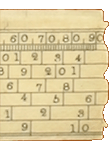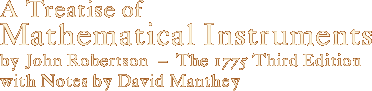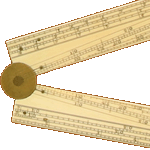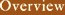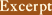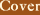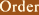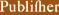# A Treatise of Mathematical Instruments

## Excerpts

The following excerpts are available:

See either the GIF or PDF file for a sample of the font and formatting used in A Treatise of Mathematical Instruments.

## From Section VII of the text.

Of the Lines of Equal Parts.

LINES of equal parts are of two sorts, viz. simply divided, and diagonally divided. Pl. V.

I. Simply divided. Draw 3 lines parallel to one another, at unequal distances, (Fig. 14.) and of any convenient length; divide this length into what number of equal parts is thought necessary, allowing some certain number of these parts to an inch, such as 2, 2 1/2, 3, 3 1/2, 4, 4 1/2, &c. which divisions distinguish by lines drawn across the three parallels. Divide the left hand division into 10 equal parts, which distinguish by lines drawn across the lower parallels only; but, for distinction sake, let the 5th division be somewhat longer than the others: and it may not be inconvenient to divide the same left-hand division into 12 equal parts, which are laid down on the upper parallel line, having the 3d, 6th, and 9th divisions distinguished by longer strokes than the rest, whereof that at the 6th make the longest.

There are, for the most part, several of these simply divided scales put on rulers, one above the other, with numbers on the left hand, shewing in each scale, how many equal parts an inch is divided into; such as 20, 25, 30, 35, 40, 45, &c. and are severally used, as the plan to be expressed should be larger or smaller.

The use of these lines of equal parts, is to lay down any line expressed by a number of two places or denominations, whether decimally, or duodecimally divided; as leagues, miles, chains, poles, yards, feet, inches, &c. and their tenth parts, or twelfth parts: thus, if each of the divisions be reckoned 1, as 1 league, mile, chain, &c. then each of the subdivisions will express 1/10 part thereof; and if each of the large divisions be called 10, then each small one will be 1: and if the large divisions be 100, then each small one will be 10, &c.

Therefore, to lay off a line 8 7/10, 87, or 870 parts, let them be leagues, miles, chains, &c. set one point of the compasses on the 8th of the large divisions, counting from the left hand towards the right, and open the compasses, till the other point falls on the 7th of the small divisions, counting from the right hand towards the left, then are the compasses opened to express a line of 8 7/10, 87 or 870 leagues, miles, chains, &c. and bears such proportion in the plan, as the line measured does to the thing represented.

But if the length of feet and inches was to be expressed, the same large divisions may represent the feet, but the inches must be taken from the upper part of the first division, which (as before noted) is divided into 12 equal parts.

Thus, if a line 7 feet 5 inches was to be laid down; set one point of the compasses on the 5th division among the 12, counting from the right hand towards the left, and extend the other to 7, among the large divisions, and that distance laid down in the plan, shall express a line of 7 feet 5 inches: and the like is to be understood of any other dimensions.

II. Diagonally divided. Draw eleven lines parallel to each other, and at equal distances; divide the upper of these lines into such a number of equal parts as the scale to be expressed is intended to contain; and from each of these divisions draw perpendiculars through the eleven parallels, (Fig. 15) subdivide the first of these divisions into 10 equal parts, both in the upper and lower lines; then each of these subdivisions may also be subdivided into 10 equal parts, by drawing diagonal lines; viz. from the 10th below, to the 9th above; from the 9th below to the 8th above; from the 8th below to the 7th above, &c. till from the 1st below to the 0th above, so that by these means one of the primary divisions on the scale, will be divided into 100 equal parts.

There are generally two diagonal scales laid on the same plane or face of the ruler, one being commonly half the other. (Fig. 15.)

The use of the diagonal scale is much the same with the simple scale; all the difference is, that a plan may be laid down more accurately by it: because in this, a line may be taken of three denominations; whereas from the former, only two could be taken.

Now from this construction it is plain, if each of the primary divisions represent 1, each of the first subdivisions will express 1/10 of 1; and each of the second subdivisions, (which are taken on the diagonal lines, counting from the top downwards) will express 1/10 of the former subdivisions, or a 100th of the primary divisions; and if each of the primary divisions express 10, the each of the first subdivisions will express 1, and each of the 2d, 1/10; and if each of the primary divisions represent 100, then each of the first subdivisions will be 10; and each of the 2d will be 1, &c.

Therefore to lay down a line, whose length is express’d by 347, 34 7/10 or 3 47/100 whether leagues, miles, chain, &c.

On the diagonal line, joined to the 4th of the first subdivisions, count 7 downwards, reckoning the distance of each parallel 1; there set one point of the compasses, and extend the other, till it falls on the intersection of the third primary division with the same parallel in which the other foot rests, and the compasses will then be opened to express a line of 347, 34 7/10, or 3 47/100, &c.

Those who have frequent occasion to use the scales, will perhaps find, that a ruler with the 20 following scales on it, viz. 10 on each face, will suit more purposes than any set of simply divided scales hitherto made public, on one ruler.

One Side } The divisions to an inch { 10,11,12,13 1/2,15,16 1/2,18,20,22,25.
Other Side -- 28,32,36,40,45,50,60,70,85,100.

The left hand primary division, to be divided into 10 and 12 and 8 parts; for these subdivisions are of great use in drawing the parts of a fortress, and of a piece of cannon.

It will here be convenient to shew, how any plan expressed by right lines and angles, may be delineated by the scales of equal parts, and the protractor.

A Treatise of Mathematical Instruments · by John Robertson · with Notes by David Manthey
ISBN 1-931468-11-7 · Copyright © 2002 by David Manthey · 6x9", 284 pages.